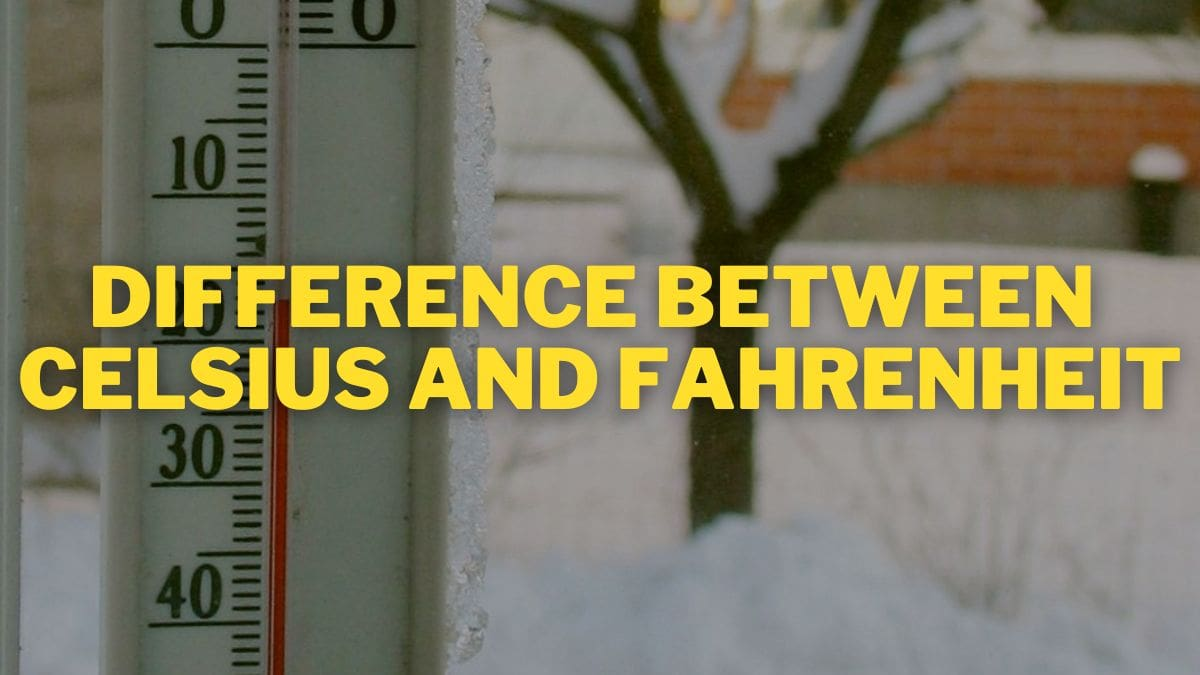# What Is The Difference Between Celsius And Fahrenheit?

Though there are a number of units to measure temperature, the most popular units to measure temperature are Celsius and Fahrenheit. There's often a wave of dilemma while one is planning to measure temperature, the question of Celsius vs Fahrenheit always arises. Find out what is the difference between the two and which scale can be used in what scenario here!What Is The Difference Between Celsius And Fahrenheit?

There's often a wave of dilemma while one is planning to measure temperature, the question of Celsius vs Fahrenheit always arises. Find out what is the difference between the two and which scale can be used in what scenario.

While every nation across the globe prefers the celsius scale it is a few countries like the United States that use Fahrenheit on a day-to-day basis.

Both scales are based on boiling and freezing points of water, though the kind of water is not the same.

To make it simple, the temperature is the measure of the degree of hotness or coldness and temperature is a physical quantity that is measurable.

It is Celsius and Fahrenheit that are the commonly used units to measure temperature.

ALSO READ: What is the Difference Between Bison and Buffalo?

## What are the Units of Temperature?

• Though there are a number of units to measure temperature, the most popular units to measure temperature are Celsius and Fahrenheit.
• Both the units have separate values for boiling and freezing points, in relation to water.

## What is Celsius?

• Celsius is also called centigrade and is used by most countries.
• It is named after a Swedish Astronomer called Andres Celsius.
• Previously known as the centigrade scale, Celsius has the symbol: °C.
• It can be referred to as a specific temperature on the Celsius scale as well as serve as a unit increment to indicate a difference between two temperatures or an uncertainty also called a temperature interval.

## What is Fahrenheit?

• Most commonly used in the United States for measuring temperature The Fahrenheit system is used as a standard for non-scientific uses.
• Named after a German-Dutch Physicist, Daniel Gabriel Fahrenheit.
• The freezing point of water is 32 degrees Fahrenheit, written as  “32 °F” in the Fahrenheit scale while the boiling point is 212 degrees.
• The boiling and freezing points of water are exactly 180 degrees apart.

## Celsius And Fahrenheit: Key Differences

 Basis of Comparison Celsius Fahrenheit Meaning It is the part of the metric system used to measure the temperature. Another part of the metric system and is used to measure the temperature. Used In Majority of the countries Only a few countries like the U.S. Year of Discovery Mid-1700 1724 Proposed By Astronomer Anders Celsius Physicist Daniel Gabriel Fahrenheit Denoted By Degree C (°C) Degree F (°F) Melting Point of Ice O°C 32°F Boiling Point of Water 99.9839°C or 100°C 211.97102 or 212°F Absolute Zero Value -273.15°C -459.67°F Average Human Body Temperature 37°C 98.6°F Conversion Can be converted into Fahrenheit by: (°C × 1.8) + 32 = °F Can be converted into Celsius by: (°F – 32) + 1.8 = °C

## Temperature Differences Between Celsius And Fahrenheit

 Temperature in Celsius Fahrenheit Absolute Zero -273.15 -459.67 Boiling Point of Water 99.9839 211.97102 Melting Point of Ice 0 32 Average Human Body Temperature 37.0 98.6 Surface of the Sun 5526 9980 Highest recorded surface temperature on the Earth 58 136.4 Lowest recorded surface temperature on the Earth -89 -128.2

## Differences in Usage

• The Fahrenheit system continues to be the accepted standard for non-scientific use in the United States.
• All across the globe, countries have adopted Celsius as the primary scale in use.
•  Fahrenheit is sometimes used for the measurement of higher temperatures.

## Which is better?

Though Celsius is used by almost every country across the globe except for the United States, its territories, and a few other small nations, the scientific community uses Celsius for many practices.

Celsius is based around 0 degrees for freezing and 100 degrees for boiling and are more straightforward, While Fahrenheit has the benefit of being more precise.

For example,

• 32 degrees Fahrenheit = 0 degrees Celsius
• 35 degrees Fahrenheit = 1.6 degrees Celsius

## Relationship with the Kelvin scale

• K = (°F + 459.67) ÷ 1.8
• °F = (K × 9/5) − 459.67
• K = °C + 273.15
• °C = K − 273.15

ALSO READ: List Of Top Ten Coldest Places In India

## FAQ

### What is temperature?

Temperature is the measure of the degree of hotness or coldness.

### What is the unit to measure the temperature?

Celsius and Fahrenheit are the units to measure the temperature.

### Who invented the Fahrenheit system?

The Fahrenheit system was introduced by Daniel Gabriel Fahrenheit.
Get the latest General Knowledge and Current Affairs from all over India and world for all competitive exams.
खेलें हर किस्म के रोमांच से भरपूर गेम्स सिर्फ़ जागरण प्ले पर